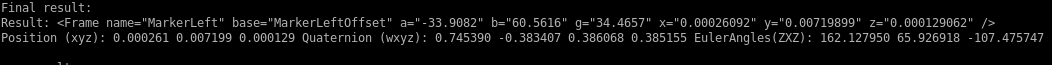[Documentation] [TitleIndex] [WordIndex]

Show EOL distros:

Package Summary

This package calculates the best approximation for a kinematic chain's parameters using rosenbrock optimization. For calculation, a layout of the kinematic chain must be provided, as well as a set of transformations between two frames of the chain that has been acquired during calibration.

Package Summary

This package calculates the best approximation for a kinematic chain's parameters using rosenbrock optimization. For calculation, a layout of the kinematic chain must be provided, as well as a set of transformations between two frames of the chain that has been acquired during calibration.

Package Summary

This package calculates the best approximation for a kinematic chain's parameters using rosenbrock optimization. For calculation, a layout of the kinematic chain must be provided, as well as a set of transformations between two frames of the chain that has been acquired during calibration.Description

This package provides a toolkit to optimize an arbitrary kinematic chain with the help of a set of calibration data.

Functionality

This package calculates the best approximation for a kinematic chain's parameters using rosenbrock optimization. For calculation, a layout of the kinematic chain must be provided, as well as a set of transformations between two frames of the chain that has been acquired during calibration. It can be used for defining new kinematic chains as well as optimizing existing. With the calculated Transformation, it is easy to define static_transformation_publisher or an urdf-file.

Usage

This package is a standalone tool for optimizing a kinematic chain. Therefore an pseudo tf-link is added to an existing kinematic chain to close it and to get a circular topology. Furthermore, offset-links are added to the kinematic chain to get some TFs to vary. A lot of training n-tuples are recorded with n=(number of non-fix joints in kinematic chain). The algorithm tries to find a kinematic chain which satisfy the configuration with all n-tuples best. An overview about the frame-file in xml-format is given now:

Frame-file

To specify an input-file use the <Data>-tag. This surrounds the whole file.

To specify the structure of your kinematic chain use the <Frames>-tag. In this area the tf-frames are defined. There are some different types of frames:

A simple parent-child relation is defined as <Frame name="Frame2" base="Frame1"/>, with Frame1 is the parent node of Frame 2.

A fixed transformation between parent and child is defined as follows

<Frame name="Frame2" base="Frame1" x="0.1" y="0.1" z="0.1" qx="1" qy="0" qz="0" qw="0"/>.

To tell the algorithm which frame can be used for optimization, boundaries are set:

<Frame name="Frame2" base="Frame1" max_x="0.3" min_y="-0.3" max_y="0.3" min_z="-0.3" max_z="0.3" min_ry="-3.0" max_ry="3.0" min_rx="-3.0" max_rx="3.0" min_rz="-3.0" max_rz="3.0"/>

Non-fixed frames are defined with parameters. These parameters are given to the optimizer within an extra file. To define a parameter, simply use square-brackets and count upwards starting at 1. Add data="true":

<Frame name="Frame2" base="Frame1" qx="()" qy="()" qz="()" qw="()" x="()" y="()" z="()" data="true"/>

This is all need to specify a kinematic chain.

To specify the goal function (for closing you kinematic chain) use the <Goal>-tag. The goal can be ether the position, orientation or both.

The position use the <Position first="Frame1" second="Frame2" />-Tag. With this goal, the algorithm tries to set the position of Frame1 equal to the one of Frame2. Same for <Orientation first="Frame1" second="Frame2" /> with the frames orientations.

A sample frame-file is given in the tutorial section.

Data-file

The data-file contains just the n-tuple datasets. One n-tuple is in one line. The single values are separated with a semicolon and a space. The program maps the data in column one to the first parameter (e. g. "") and so on.Result

The optimizer outputs the calculated values for the kinematic chain parameters directly to the console.The line "Result" shows the optimized frames which were specified in the kinematic chain.

Needed packages

Needed hardware

No hardware is needed. But it is recommended to have a real robot or construction to get some real training-data.

Start system

roslaunch asr_kinematic_chain_optimizer optimizer.launch

Don't forget to set args!

ROS Nodes

Parameters

The following args can be passed to the program:

• -h [ --help]: show help screen
• --exit: show only initial error
• --filename arg (=data/calib_dump): load data from filename (data-file)
• --initial arg: Initial value for optimization, e.g. 0.6 0.4 0.3 (n = 3)
• --cfg arg (=data/frames.xml): load cfg from filename (frame-file)
• --debug arg (=5): debug level
• --trim arg (=0.20000000000000001): trimming percentage
• --epsilon arg (=1e-08): convergence limit, 0.00000001
• --random arg (=0): use random start value
• --always arg (=0): always show result
• --max arg (=10000000): use max number of data
• --iterations arg (=500): use max number of iterations
• --debugiterations arg (=100): show result each x iterations
• --ivlinewidth arg (=0.5): width of Inventor line
• --ivcoordscale arg (=0.20000000000000001): scaleFactor of Inventor coordinatesystem

Tutorials

<Data>

• <Frames>

• <Frame name="base_link" base="" x="0" y="0" z="0" /> <Frame name="base_laser" base="base_link" x="0.27" y="0" z="0.22" /> <Frame name="ptu_pan_link" base="base_link" x="0.0" y="0.0" z="1.35" min_x="-0.3" max_x="0.3" min_y="-0.5" max_y="-0.1" min_z="1.0" max_z="1.5" min_rx="-4.0" max_rx="4.0" min_ry="-4.0" max_ry="4.0" min_rz="-4.0" max_rz="4.0" /> <Frame name="PanRotated" base="ptu_pan_link" x="0" y="0" z="0" a="()*0.01745329251" data="true"/> <Frame name="TILTBase" base="PanRotated" x="0" y="0" z="0" min_x="-0.1" max_x="0.1" min_y="-0.1" max_y="0.1" min_z="-0.1" max_z="0.1" min_rx="-4.0" max_rx="4.0" min_ry="-4.0" max_ry="4.0" min_rz="-4.0" max_rz="4.0" /> <Frame name="TILTRotated" base="TILTBase" x="0" y="0" z="0" g="()*0.01745329251" /> <Frame name="PTU" base="TILTRotated" x="0" y="0" z="0" min_x="-0.1" max_x="0.1" min_y="-0.1" max_y="0.1" min_z="-0.1" max_z="0.1" min_rx="-4.0" max_rx="4.0" min_ry="-4.0" max_ry="4.0" min_rz="-4.0" max_rz="4.0" /> <Frame name="PTUOffset" base="base_laser" qx="()" qy="()" qz="()" qw="()" x="()" y="()" z="()" data="true"/>

</Frames> <Goal>

• <Position first="PTU" second="PTUOffset"/> <Orientation first="PTU" second="PTUOffset"/>

</Goal>

</Data>

2022-01-08 12:28